l

####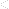BACK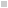HomeHistory WingAdventure WingExhibits & ProgramsCompany StoreInformation Desk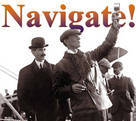###The Wright     TriaNgle(You are here.)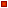###Crunching the Numbers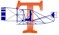he key that unlocked the secret of flight was a triangle – specifically, a right triangle. That is, a triangle with one "right" or 90 degree (°) angle. Over three thousand years ago, Babylonian astronomers found that by using right triangles they could predict the motions of the sun and moon. About 160 BCE, the Greek astronomer Hipparchos organized these predictive methods into a mathematic system. Later Arabic astronomers shaped it into an entire branch of mathematics that became known as trigonometry. It was first used exclusively to solve problems in astronomy, but scholars quickly found that it also had applications in geometry, navigation, architecture, and by Orville and Wilbur's time, physics. Wilbur, in fact, took a college-prep course in trigonometry while he was still thinking of attending Yale.

## A Trig Primer

It's a dead-simple system. At its heart is a single precept: If you add the angles at the corners of a triangle, you always get 180° (Figure 1). In a right triangle, you know that one corner is always 90°. Since 180 minus 90 equals 90, the other two corners or complimentary corners, must total 90°. So if you know the angle of just one complimentary corner, you can always find the angle of the other simply by subtracting the angle you know from 90°. For example, if one of the complimentary angles in a right triangle is 60°, the other must be 30°: 90 - 60 = 30 (Figure 2).

Hipparchos took this a step further. He knew that the shape of a right triangle is determined by its complimentary angles. And as long as those angles don't change, this shape always remains proportionate no matter what the size of the triangle. Another way to say it is that for any one set of angles, the sides of a right triangle are always the same ratios to one another. If you know these ratios, and you know the length of one part, you can use the ratios to find the length of the other parts. For example, if you know that one side of a right triangle is half as long as another (a ratio of 1 to 2, or 1/2), and you know that the longer side is 10 inches long, then it is a simple matter to figure that the shorter side is 5 inches: 1/2 x 10 = 5.

Because the shape of the triangle varies with different complimentary angles, so do the ratios. In fact, there are a different set of ratios for every angle between 0° and 90° and each angle has three major ratios called the sine, cosine, and tangent. These ratios are figured by dividing the length of one side into another. Which side gets divided by what is determined by the position of the sides in relation to the complimentary angle to which the ratio belongs. The side opposite the angle is the opposite side. The remaining side, the one that is part of the complimentary angle, is the adjacent side. The side immediately opposite the right angle is always the hypotenuse. The angle itself is often represented by the Greek letter theta or Θ (Figure 3).

• The sine of an angle (Θ) is the opposite side divided by the hypotenuse:  sinΘ = opposite/hypotenuse
• The cosine of angle Θ is the adjacent side divided by the hypotenuse: cosΘ = adjacent/hypotenuse
• The tangent of angle Θ is the opposite side divided by the adjacent: tanΘ = opposite/adjacent.

Hipparchos gathered the ratios for each angle into lists or tables of sines, cosines, and tangents. With these tables, he could calculate the length of any side of a right triangle as long as he knew one complimentary angle and the length of one other side. For example, if he needed to find the length of the opposite side of a triangle where the known complimentary angle was 30° and the length of the hypotenuse was 5 inches, all he had to do was look up the sine of 30° and multiply it times 5. The sine of 30° is 0.5: 0.5 x 5 = 2.5 (Figure 4).

And that is the most important idea to take away from this overview. Even if you didn't understand all that stuff about ratios, remember that all you need to know is one angle and one measurement to discover all the remaining angles and measurements of a right triangle. Early aeronautics was built on that simple premise.

## Measuring Lift and Drift

Early in the nineteenth century, Sir George Cayley defined the forces of an airplane in flight. The lift generated by the wings must overcome gravity; thrust must overcome drag to propel the aircraft through the air. The sketches in Cayley's notebooks and publications show the forces of lift and drag acting perpendicular to one another. One of his earliest drawings, etched on a silver disc in 1799, represents lift and drag as the sides of a right triangle.

Cayley referred to drag as "resistance," the Wright brothers called it "drift." They could see the results of lift and drift every time they flew one of their scientific kites. The vertical distance of the kite above the ground was due to lift, while the horizontal distance from the operator to the kite was due to drift. And the hypotenuse of the lift-drift triangle was traced by the tether string of the kite.

At some point in his kite experiments, it occurred to Wilbur that the tension on the tether was the direct result of the combined forces of lift and drift. If he measured that tension and the angle of the tether above the horizon, he would have one complimentary angle and the magnitude of the hypotenuse formed by these forces. And he could use these measurements, along with a table of sines and cosines, to find the precise lift and drift acting on the kite as it flew.

This is why the Wrights flew their gliders as kites at Kitty Hawk. Using an inclinometer, they measured the angle of the tether lines. They measured the tension on these lines with a spring scale, such as the fishermen of Kitty Hawk used to weigh their catch. They also used the scale to weigh the glider. The Wrights needed the glider weight to compensate for another force in play – gravity. The lift-drag triangle manifested by the glider in flight actually revealed the excess lift, the lift over and above what was needed to overcome gravity and fly the glider. To find the total lift, the Wrights would have to add the glider weight to the excess lift.

They also borrowed an anemometer from Joseph Dosher at the Kitty Hawk Lifesaving Station to measure the wind speed. The tension on the tether lines, they knew, would vary with the wind speed. The fiercer the wind, the greater the tension. The forces of lift and drift would increase proportionately with the wind.

Once they had all these measurements, they simply applied a little trigonometry.

• The excess lift (the opposite leg of the triangle) was the sine of the angle times the tension on the tether. The total lift was the excess lift plus the weight of the glider: (sinΘ x tension) + glider weight = lift.
• The drift or drag (the adjacent side of the triangle) was the cosine of the angle times the tension: cosΘ x tension = drag.

This may have seemed like a simple exercise to Wilbur and Orville, but it was a groundbreaking development in aeronautics. For the first time, scientists measured the performance of an aircraft in flight and compared it to laboratory results – in this case, the results from Lilienthal's whirling arm experiments. In the estimation of the Wright brothers the 1900 glider  was a failure, able to support the weight of a pilot only in extremely high winds. But failed or not, the results of their experiments pointed them in the direction of eventual success.